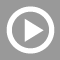#Videos

## SAT Math VideosThis is the third of three videos reviewing SAT math number properties, including integers, digits, odd-even, signed numbers, prime numbers, factors, divisors, multiples, properties of zero, rounding and remainders. 12 minutes.This is the second of three videos reviewing SAT math number properties, including integers, digits, odd-even, signed numbers, prime numbers, factors, divisors, multiples, properties of zero, rounding and remainders. 15 minutes.This is the first of three videos reviewing SAT math number properties, including integers, digits, odd-even, signed numbers, prime numbers, factors, divisors, multiples, properties of zero, rounding and remainders. 14 minutes.